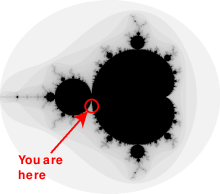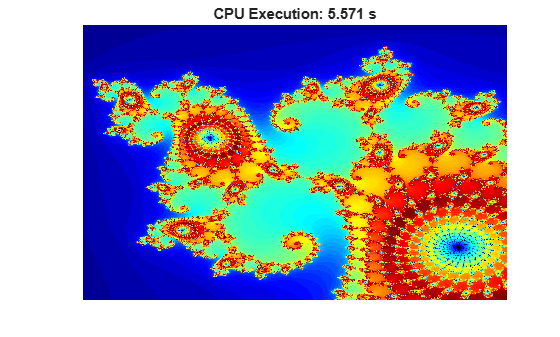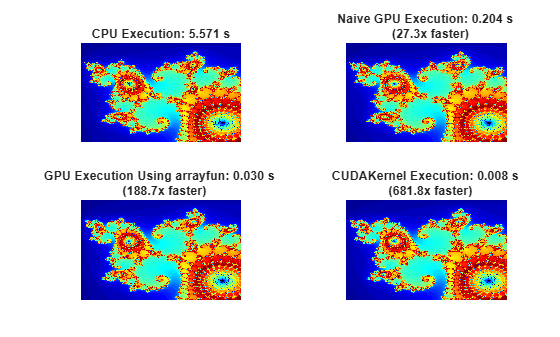# Illustrating Three Approaches to GPU Computing: The Mandelbrot Set

This example shows how to adapt your MATLAB® code to compute the Mandelbrot Set using a GPU.

Starting with an existing algorithm, this example shows how to adapt your code using Parallel Computing Toolbox™ to make use of GPU hardware in three ways:

1. Using the existing algorithm but with GPU data as input

2. Using `arrayfun` to perform the algorithm on each element independently

3. Using the MATLAB/CUDA interface to run some existing CUDA/C++ code

### Setup

The values below specify a highly zoomed part of the Mandelbrot Set in the valley between the main cardioid and the p/q bulb to its left.A 1000x1000 grid of real parts (X) and imaginary parts (Y) is created between these limits and the Mandelbrot algorithm is iterated 500 times at each grid location.

```maxIterations = 500; gridSize = 1000; xlim = [-0.748766713922161, -0.748766707771757]; ylim = [ 0.123640844894862, 0.123640851045266];```

### The Mandelbrot Set in MATLAB

Below is an implementation of the Mandelbrot Set using standard MATLAB commands running on the CPU. This is based on the code provided in Cleve Moler's Experiments with MATLAB e-book. Time the execution on the CPU using `tic` and `toc`.

Setup the two-dimensional grid of complex values.

```tic; x = linspace(xlim(1),xlim(2),gridSize); y = linspace(ylim(1),ylim(2),gridSize); [xGrid,yGrid] = meshgrid(x,y); z0 = xGrid + 1i*yGrid;```

For 500 iterations, calculate the next value of a point on the complex grid `z` by squaring the previous value and adding its initial value, `z0`. Count the number of iterations for which the magnitude of `z` is less than or equal to two. This calculation is vectorized such that every location is updated at once.

```cpuCount = ones(size(z0)); z = z0; for n = 0:maxIterations z = z.*z + z0; inside = abs(z)<=2; cpuCount = cpuCount + inside; end cpuCount = log(cpuCount); cpuTime = toc```
```cpuTime = 4.4007 ```

Plot the natural logarithm of the count.

```figure imagesc(x,y,cpuCount); c = colormap([jet;flipud(jet);0 0 0]); axis off title(sprintf("CPU Execution: %1.3f s",cpuTime));```### Using `gpuArray`

When MATLAB encounters data on the GPU, calculations with that data are performed on the GPU. The class `gpuArray` provides GPU versions of many functions that you can use to create data arrays, including the `linspace`, `logspace`, and `meshgrid` functions needed here. Similarly, the `count` array is initialized directly on the GPU using the function `ones`.

Ensure that your desired GPU is available and selected.

```gpu = gpuDevice; disp(gpu.Name + " GPU selected.")```
```NVIDIA RTX A5000 GPU selected. ```

Call the `naiveGPUMandelbrot` function. The supporting function `naiveGPUMandelbrot` applies the Mandelbrot algorithm for each point on the grid on the GPU and is provided at the end of this examples.

`[x,y,naiveGPUCount] = naiveGPUMandelbrot(xlim,ylim,gridSize,maxIterations);`

Time the execution of the function on the GPU using `gputimeit`. For functions that use the GPU, `gputimeit` is better than `tic` and `toc` or `timeit` because it ensures that all operations on the GPU finish before recording the elapsed time.

`naiveGPUTime = gputimeit(@() naiveGPUMandelbrot(xlim,ylim,gridSize,maxIterations))`
```naiveGPUTime = 0.2181 ```

### Element-wise Operation

Noting that the algorithm is operating equally on every element of the input, we can place the code in a function and call it using `arrayfun`. The function `processMandelbrotElement` is provided as a supporting function at the end of this example. For `gpuArray` inputs, the function used with `arrayfun` gets compiled into native GPU code.

An early abort has been introduced into the function `processMandelbrotElement` because this function processes only a single element. For most views of the Mandelbrot Set a significant number of elements stop very early and this can save a lot of processing. The `for`-loop has also been replaced by a `while`-loop because they are usually more efficient. This function makes no mention of the GPU and uses no GPU-specific features.

Using `arrayfun` causes MATLAB to make one call to a parallelized GPU operation that performs the whole calculation, instead of many thousands of calls to separate GPU-optimized operations (at least 6 per iteration). The first time you call `arrayfun` to run a particular function on the GPU, there is some overhead time to set up the function for GPU execution. Subsequent calls of `arrayfun` with the same function can run faster.

Setup the two-dimensional grid of complex values.

```xGrid = gpuArray(xGrid); yGrid = gpuArray(yGrid);```

Using `arrayfun`, apply the Mandelbrot algorithm for each point on the grid.

```gpuArrayfunCount = arrayfun(@processMandelbrotElement, ... xGrid,yGrid,maxIterations);```

Time the `arrayfun` execution using `gputimeit`.

```gpuArrayfunTime = gputimeit(@() arrayfun(@processMandelbrotElement, ... xGrid,yGrid,maxIterations))```
```gpuArrayfunTime = 0.0308 ```

### Working with CUDA

In Experiments in MATLAB performance is improved by converting the basic algorithm to a C-Mex function. If you are willing to do some work in C/C++, then you can use Parallel Computing Toolbox to call pre-written CUDA kernels using MATLAB data. For more details on using CUDA kernels in MATLAB, see Run CUDA or PTX Code on GPU.

A CUDA/C++ implementation of the element processing algorithm is provided with this example, `pctdemo_processMandelbrotElement.cu`. The part of the CUDA/C++ code that executes the Mandelbrot algorithm for a single location is given below.

```__device__ unsigned int doIterations( double const realPart0, double const imagPart0, unsigned int const maxIters ) { // Initialize: z = z0 double realPart = realPart0; double imagPart = imagPart0; unsigned int count = 0; // Loop until escape while ( ( count <= maxIters ) && ((realPart*realPart + imagPart*imagPart) <= 4.0) ) { ++count; // Update: z = z*z + z0; double const oldRealPart = realPart; realPart = realPart*realPart - imagPart*imagPart + realPart0; imagPart = 2.0*oldRealPart*imagPart + imagPart0; } return count; } ```

Compile this file into a parallel thread execution (PTX) file using `mexcuda`.

`mexcuda -ptx pctdemo_processMandelbrotElement.cu`
```Building with 'NVIDIA CUDA Compiler'. MEX completed successfully. ```

Create a `parallel.gpu.CUDAKernel` object by passing the CUDA file and the PTX file to the `parallel.gpu.CUDAKernel` function.

```cudaFilename = "pctdemo_processMandelbrotElement.cu"; ptxFilename = "pctdemo_processMandelbrotElement.ptx"; kernel = parallel.gpu.CUDAKernel(ptxFilename,cudaFilename);```

One GPU thread is required per location in the Mandelbrot Set, with the threads grouped into blocks. The kernel indicates how big a thread-block is. Calculate the number of thread-blocks required, and set the `GridSize` property of the kernel (effectively the number of thread blocks that will be launched independently by the GPU) accordingly.

```numElements = numel(xGrid); kernel.ThreadBlockSize = [kernel.MaxThreadsPerBlock,1,1]; kernel.GridSize = [ceil(numElements/kernel.MaxThreadsPerBlock),1];```

Evaluate the kernel using `feval`.

```count = zeros(size(xGrid),"gpuArray"); gpuCUDAKernelCount = feval(kernel,count,xGrid,yGrid,maxIterations,numElements);```

Time the kernel execution using `gputimeit`.

`gpuCUDAKernelTime = gputimeit(@() feval(kernel,count,xGrid,yGrid,maxIterations,numElements));`

### Summary

Plot the results from the different methods and compare the execution times.

```method = ["Naive GPU Execution" "GPU Execution Using arrayfun" "CUDAKernel Execution"]; count = cat(3,naiveGPUCount,gpuArrayfunCount,gpuCUDAKernelCount); time = [naiveGPUTime gpuArrayfunTime gpuCUDAKernelTime]; figure colormap(c) tiledlayout("flow") nexttile imagesc(x,y,cpuCount); axis off title(sprintf("CPU Execution: %1.3f s",cpuTime)); for idx = 1:3 nexttile imagesc(x,y,count(:,:,idx)) axis off title(sprintf("%s: %1.3f s \n (%1.1fx faster)", ... method(idx),time(idx),cpuTime/time(idx))) end```This example has shown three ways in which a MATLAB algorithm can be adapted to make use of GPU hardware:

1. Convert the input data to be on the GPU using `gpuArray`, leaving the algorithm unchanged.

2. Use `arrayfun` on a `gpuArray` input to perform the algorithm on each element of the input independently.

3. Use a `parallel.gpu.CUDAKernel` to run some existing CUDA/C++ code using MATLAB data.

The code in this example was timed on a Windows® 10, Intel® Xeon® W-2133 @ 3.60 GHz test system with an NVIDIA® RTX A5000 GPU.

### Supporting Functions

The supporting function `naiveGPUMandelbrot` creates a two-dimensional grid of complex values and counts the number of iterations before the complex value number `(x0,y0)` jumps outside a circle of radius 2 on the complex plane. Each iteration involves mapping `z = z^2 + z0` where `z0 = x0 + i*y0`. The function returns the grid coordinate vectors and the log of the iteration count at escape or `maxIterations` if the point did not escape. By initializing data on the GPU and operating on this data, the `naiveGPUMandelbrot` function executes the Mandelbrot algorithm on the GPU.

```function [x,y,count] = naiveGPUMandelbrot(xlim,ylim,gridSize,maxIterations) x = gpuArray.linspace(xlim(1),xlim(2),gridSize); y = gpuArray.linspace(ylim(1),ylim(2),gridSize); [xGrid,yGrid] = meshgrid(x,y); z0 = complex(xGrid,yGrid); count = ones(size(z0),"gpuArray"); z = z0; for n = 0:maxIterations z = z.*z + z0; inside = abs(z)<=2; count = count + inside; end count = log(count); end```

The supporting function `processMandelbrotElement` creates a two-dimensional grid of complex values and counts the number of iterations before the complex value number `(x0,y0)` jumps outside a circle of radius 2 on the complex plane. Each iteration involves mapping `z = z^2 + z0` where `z0 = x0 + i*y0`. The function returns the log of the iteration count at escape or `maxIterations` if the point did not escape.

```function count = processMandelbrotElement(x0,y0,maxIterations) z0 = complex(x0,y0); z = z0; count = 1; while (count <= maxIterations) && (abs(z) <= 2) count = count + 1; z = z*z + z0; end count = log(count); end```